# Keras Learning Rate Finder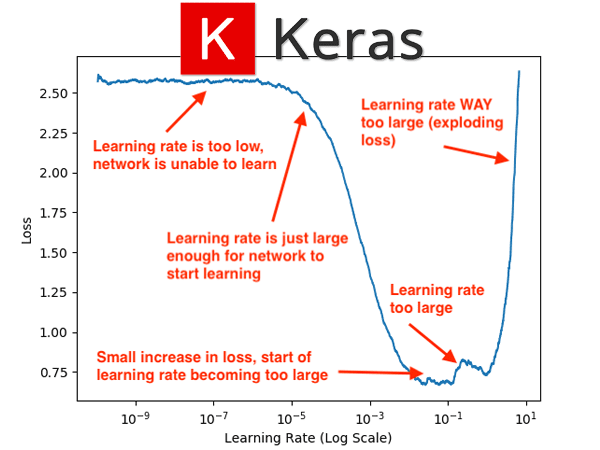In this tutorial, you will learn how to automatically find learning rates using Keras. This guide provides a Keras implementation of fast.ai’s popular “lr_find” method.

Today is part three in our three-part series of learning rate schedules, policies, and decay using Keras:

1. Part #1: Keras learning rate schedules and decay
2. Part #2: Cyclical Learning Rates with Keras and Deep Learning (last week’s post)
3. Part #3: Keras Learning Rate Finder (today’s post)

Last week we discussed Cyclical Learning Rates (CLRs) and how they can be used to obtain high accuracy models with fewer experiments and limited hyperparameter tuning.

The CLR method allows our learning rate to cyclically oscillate between a lower and upper bound; however, the question still remains, how do we know what are good choices for our learning rates?

Today I’ll be answering that question.

And by the time you have completed this tutorial, you will understand how to automatically find optimal learning rates for your neural network, saving you 10s, 100s or even 1000s of hours in compute time running experiments to tune your hyperparameters.

To learn how to find optimal learning rates using Keras, just keep reading!

Looking for the source code to this post?

## Keras Learning Rate Finder

In the first part of this tutorial we’ll briefly discuss a simple, yet elegant, algorithm that can be used to automatically find optimal learning rates for your deep neural network.

From there, I’ll show you how to implement this method using the Keras deep learning framework.

We’ll apply the learning rate finder implementation to an example dataset, enabling us to obtain our optimal learning rates.

We’ll then take the found learning rates and fully train our network using a Cyclical Learning Rate policy.

### How to automatically find optimal learning rates for your neural network architecture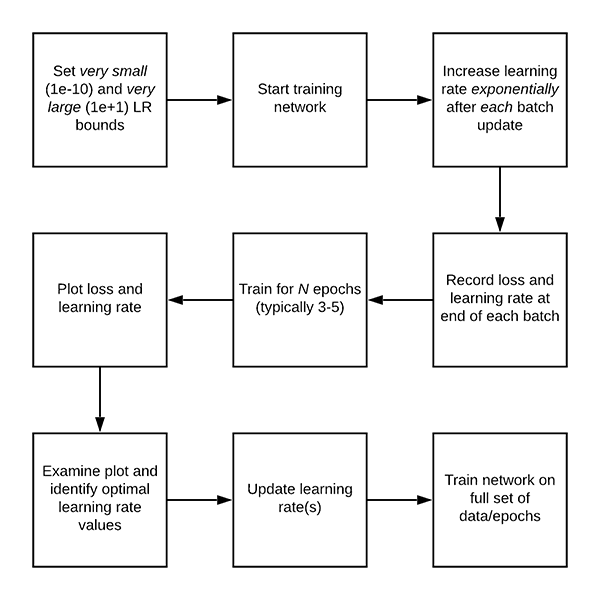Figure 1: Deep learning requires tuning of hyperparameters such as the learning rate. Using a learning rate finder in Keras, we can automatically find a suitable min/max learning rate for cyclical learning rate scheduling and apply it with success to our experiments. This figure contains the basic algorithm for a learning rate finder.

In last week’s tutorial on Cyclical Learning Rates (CLRs), we discussed Leslie Smith’s 2017 paper, Cyclical Learning Rates for Training Neural Networks.

Based on the title of the paper alone, the obvious contribution to the deep learning community by Dr. Smith is the Cyclical Learning Rate algorithm.

However, there is a second contribution that is arguably more important than CLRs — automatically finding learning rates. Inside the paper, Dr. Smith proposes a simple, yet elegant solution on how we can automatically find optimal learning rates for training.

Note: While the algorithm was introduced by Dr. Smith, it wasn’t popularized until Jermey Howard of fast.ai suggested that his students use it. If you’re a fast.ai user, you may remember the learn.lr_find  function — we’ll be implementing a similar method using Keras instead.

The automatic learning rate finder algorithm works like this:

• Step #1: We start by defining an upper and lower bound on our learning rate. The lower bound should be very small (1e-10) and the upper bound should be very large (1e+1).
• At 1e-10 the learning rate will be too small for our network to learn, while at 1e+1 the learning rate will be too large and our model will overfit.
• Both of these are okay, and in fact, that’s what we hope to see!
• Step #2: We then start training our network, starting at the lower bound.
• After each batch update, we exponentially increase our learning rate.
• We log the loss after each batch update as well.
• Step #3: Training continues, and therefore the learning rate continues to increase until we hit our maximum learning rate value.
• Typically, this entire training process/learning rate increase only takes 1-5 epochs.
• Step #4: After training is complete we plot a smoothed loss over time, enabling us to see when the learning rate is both:
• Just large enough for loss to decrease
• And too large, to the point where loss starts to increase.

The following figure (which is the same as the header at the very top of the post, but included here again so you can easily follow along) visualizes the output of the learning rate finder algorithm on the CIFAR-10 dataset using a variation of GoogLeNet (which we’ll utilize later in this tutorial):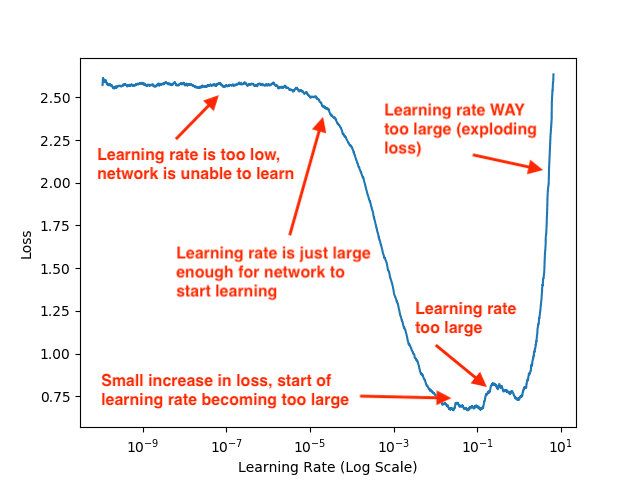Figure 2: When inspecting a deep learning experiment, be sure to look at the loss landscape. This plot helps you identify when your learning rate is too low or too high.

While examining this plot, keep in mind that our learning rate is exponentially increasing after each batch update. After a given batch completes, we increase the learning rate for the next batch.

Notice that from 1e-10 to 1e-6 our loss is essentially flat — the learning rate is too small for the network to actually learn anything. Starting at approximately 1e-5 our loss starts to decline — this is the smallest learning rate where our network can actually learn.

By the time we hit 1e-4 our network is learning very quickly. At a little past 1e-2 there is a small increase in loss, but the big increase doesn’t begin until 1e-1.

Finally, by 1e+1 our loss has exploded — the learning rate is far too high for our model to learn.

Given this plot, I can visually examine it and pick out my lower and upper bounds on my learning rate for CLR:

• Lower bound: 1e-5
• Upper bound: 1e-2

If you are using a learning rate schedule/decay policy then you would select 1e-2 as your initial learning rate and then decrease it as you train.

However, in this tutorial we’ll be using a Cyclical Learning Rate policy so we need both the lower and upper bound.

But, the question still remains:

How did I generate that learning rate plot?

I’ll be answering that question later in this tutorial.

### Project structure

Go ahead and grab the “Downloads” for today’s post which contain last week’s CLR implementation and this week’s Learning Rate Finder in addition to our training script.

Then, inspect the project layout with the tree  command:

Our output/  directory contains three plots:

• The Cyclical Learning Rate graph.
• The Learning Rate Finder loss vs. learning rate plot.
• Our training accuracy/loss history plot.

The pyimagesearch module contains three classes and a configuration:

• clr_callback.py : Contains the CyclicLR  class which is implemented as a Keras callback.
• learningratefinder.py : Holds the LearningRateFinder  class — the focus of today’s tutorial.
• minigooglenet.py : Has our MiniGoogLeNet CNN class. We won’t go into details today. Please refer to Deep Learning for Computer Vision with Python for a deep dive into GoogLeNet as well as MiniGoogLeNet.
• config.py : A Python file which holds configuration parameters/variables.

After we review the config.py  and learningratefinder.py  files, we’ll walk through train.py , our Keras CNN training script.

### Implementing our automatic Keras learning rate finder

Let’s now define a class named LearningRateFinder  that encapsulates the algorithm logic from the “How to automatically find optimal learning rates for your neural network architecture” section above.

Our implementation is inspired by the LRFinder  class from the ktrain library — my primary contribution is a few adjustments to the algorithm, including detailed documentation and comments for the source code, making it easier for readers to understand how the algorithm works.

Let’s go ahead and get started — open up the learningratefinder.py  file in your project directory and insert the following code:

Packages are imported on Lines 2-6. We’ll use a LambdaCallback  to instruct our callback to run at the end of each batch update. We will use matplotlib  to implement a method called plot_loss  which plots the loss vs. learning rate.

The constructor to the LearningRateFinder  class begins on Line 9.

First, we store the initialized model, stopFactor which indicates when to bail out of training (if our loss becomes too large), and beta  value (used for averaging/smoothing our loss for visualization purposes).

We then initialize our lists of learning rates along with the loss values for the respective learning rates (Lines 17 and 18).

We proceed to perform a few more initializations, including:

• lrMult: The learning rate multiplication factor.
• avgLoss: Average loss over time.
• bestLoss: The best loss we have found when training.
• batchNum: The current batch number update.
• weightsFile: Our path to the initial model weights (so we can reload the weights and set them to their initial values prior to training after our learning rate has been found).

The reset  method is a convenience/helper function used to reset all variables from our constructor:

When using our LearningRateFinder  class, must be able to work with both:

• Data that can fit into memory.
• Data that either (1) is loaded via a data generator and is therefore too large to fit into memory or (2) needs data augmentation/additional processing applied to it and thus uses a generator.

If the entire dataset can fit into memory and no data augmentation is applied, we can use Keras’ .fit method to train our model.

However, if we are using a data generator, either out of memory necessity or because we are applying data augmentation, we instead need to use Keras’ .fit_generator function for training.

Note: You can read about the differences between .fit and .fit_generator in this tutorial.

The is_data_generator function can determine if our input data is a raw NumPy array or if we are using a data generator:

Here we are checking to see if the name of the input data class belongs to the set of data iterator classes defined in Lines 41 and 42.

If you are using your own custom data generator simply encapsulate it in a class and then add your class name to the list of iterClasses.

The on_batch_end  function is responsible for updating our learning rate after every batch is complete (i.e., both the forward and backward pass):

We’ll be using this method as a Keras callback and therefore we need to ensure our function accepts two variables Keras expects to see — batch  and logs.

Lines 50 and 51 grab the current learning rate from our optimizer and then add it to our learning rates list ( lrs ).

Lines 57 and 58 grab the loss at the end of the batch and then increment our batch number.

Lines 58-61 compute the average loss, smooth it, and then update the losses list with the smooth average.

Line 64 computes our maximum loss value which is a function of our stopFactor and our bestLoss found thus far.

If our smoothLoss has grown to be larger than our stopLoss, then we stop training (Lines 67-70).

Lines 73 and 74 check to see if a new bestLoss  has been found, and if so, we update the variable.

Finally, Line 77 increases our learning rate while Line 78 sets the learning rate value for the next batch.

Our next method, find, is responsible for automatically finding our optimal learning rate for training. We’ll call this method from our driver script when we are ready to find our learning rate:

Our find  method accepts a number of parameters, including:

• trainData: Our training data (either a NumPy array of data or a data generator).
• startLR: The initial, starting learning rate.
• epochs: The number of epochs to train for (if no value is supplied we’ll compute the number of epochs).
• stepsPerEpoch: The total number of batch update steps per each epoch.
• batchSize: The batch size of our optimizer.
• sampleSize: The number of samples from trainData to use when finding the optimal learning rate.
• verbose: Verbosity setting for Keras .fit and .fit_generator functions.

Line 84 resets our class-specific variables while Line 87 checks to see if we are using a data iterator or a raw NumPy array.

In the case that we are using a data generator and there is no stepsPerEpoch variable supplied, we raise an exception since we cannot possibly determine the stepsPerEpoch from a generator (see this tutorial for more information on why this is true).

Otherwise, if we are not using a data generator we grab the numSamples from the trainData and then compute the number of stepsPerEpoch by dividing the number of data points by our batchSize (Lines 97-101).

Finally, if no number of epochs were supplied, we compute the number of training epochs by dividing the sampleSize by the number of stepsPerEpoch. My personal preference is to have the number of epochs ultimately be in the 3-5 range — long enough to obtain reliable results but not so long that I waste hours and hours of training time.

Let’s move on to the heart of the automatic learning rate finder algorithm:

Line 111 computes the total number of batch updates that will take place when finding our learning rate.

Using the numBatchUpdates we deriving the learning rate multiplication factor ( lrMult) which is used to exponentially increase our learning rate (Line 116).

Lines 121 and 122 create a temporary file to store our model’s initial weights. We’ll then restore these weights when our learning rate finder has completed running.

Next, we grab the original learning rate for the optimizer (Line 126), store it in origLR , and then instruct Keras to set the initial learning rate ( startLR ) for the optimizer.

Let’s create our LambdaCallback which will call our on_batch_end method each time a batch completes:

Lines 132 and 133 construct our callback — each time a batch completes the on_batch_end method will be called to automatically update our learning rate.

In the event we’re using a data generator, we’ll train our model using the .fit_generator  method (Lines 136-142). Otherwise, our entire training data exists in memory as a NumPy array, so we can use the .fit method (Lines 145- 152).

After training is complete, we reset the initial model weights and learning rate value (Lines 155 and 156).

Our final method, plot_loss, is used to plot both our learning rates and losses over time:

This exact method generated the plot you saw in Figure 2.

Later, in the “Finding our optimal learning rate with Keras” section of this tutorial, you’ll discover how to use the LearningRateFinder  class we just implemented to automatically find optimal learning rates with Keras.

### Our configuration file

Before we implement our actual training script, let’s create our configuration.

Open up the config.py file and insert the following code:

We’ll be using the Fashion MNIST as the dataset for this project. Lines 5 and 6 set the class labels for the Fashion MNIST dataset.

Our Cyclical Learning Rate parameters are specified on Lines 10-15. The MIN_LR and MAX_LR will be found in the “Finding our optimal learning rate with Keras” section below, but we’re including them here as a matter of completeness. If you need to review these parameters, please refer to last week’s post.

We will output three types of plots and the paths are specified via Lines 19-21. Two of the plots will be the same format as last week’s (training and CLR). The new type is the “learning rate finder” plot.

### Implementing our learning rate finder training script

Our learning rate finder script will be responsible for both:

1. Automatically finding our initial learning rate using the LearningRateFinder  class we implemented earlier in this guide
2. And taking the learning rate values we find and then training the network on the entire dataset.

Let’s go ahead and get started!

Open up the train.py file and insert the following code:

Lines 2-19 import our required packages. Notice how we import our LearningRateFinder  as well as our CyclicLR  callback. We’ll be training MiniGoogLeNet  on fashion_mnist . To learn more about the dataset, be sure to read Fashion MNIST with Keras and Deep Learning.

Let’s go ahead and parse a command line argument:

We have a single command line argument, --lr-find , a flag which indicates whether or not to find the optimal learning rate.

Next, let’s prepare our data:

Here we:

• Load the Fashion MNIST dataset (Line 29).
• Resize each image from 28×28 images to 32×32 images (what our network expects the inputs to be) on Lines 33 and 34.
• Scale pixel intensities to the range [0, 1] (Lines 37 and 38).
• Binarize labels (Lines 46-48).
• Construct our data augmentation object (Lines 51-53). Read more about data augmentation in my previous posts as well as in the Practitioner Bundle of Deep Learning for Computer Vision with Python.

From here, we can compile  our model :

Our model  is compiled with SGD  (Stochastic Gradient Descent) optimization. We use "categorical_crossentropy"  loss since we have > 2 classes. Be sure to use "binary_crossentropy"  if your dataset only has 2 classes.

The following if-then block handles the case when we’re finding the optimal learning rate:

Line 64 checks to see if we should attempt to find optimal learning rates. Assuming so, we:

• Initialize LearningRateFinder  (Line 68).
• Start training with a 1e-10  learning rate and exponentially increase it until we hit 1e+1  (Lines 69-73).
• Plot the loss vs. learning rate and save the resulting figure (Lines 77 and 78).
• Gracefully exit the script after printing a couple of messages to the user (Lines 83-85).

After this code executes we now need to:

1. Review the generated plot.
2. Update config.py with our MIN_LR  and MAX_LR, respectively.
3. Train the network on our full dataset.

Assuming we have completed steps 1 and 2, now let’s handle the 3rd step where our minimum and maximum learning rate have already been found and updated in the config. In this case, it is time to initialize our Cyclical Learning Rate class and commence training:

Our CyclicLR  is initialized with the freshly set parameters in our config file (Lines 90-95).

Then our model  is trained using `.fit_generator` with our aug  data augmentation object and our clr  callback (Lines 99-105).

Upon training completion, we proceed to evaluate our network on the testing set (Line 109). A classification_report  is printed in the terminal for us to inspect.

Finally, let’s plot both our training history and CLR history:

Two plots are generated for the training procedure to accompany the learning rate finder plot that we already should have:

• Training accuracy/loss history (Lines 114-125). The standard plot format included in most of my tutorials and every experiment of my deep learning book.
• Learning rate history (Lines 128-134). This plot will help us to visually verify that our learning rate is oscillating according to our CLR intentions.

### Finding our optimal learning rate with Keras

We are now ready to find our optimal learning rates!

Make sure you’ve used the “Downloads” section of the tutorial to download the source code — from there, open up a terminal and execute the following command: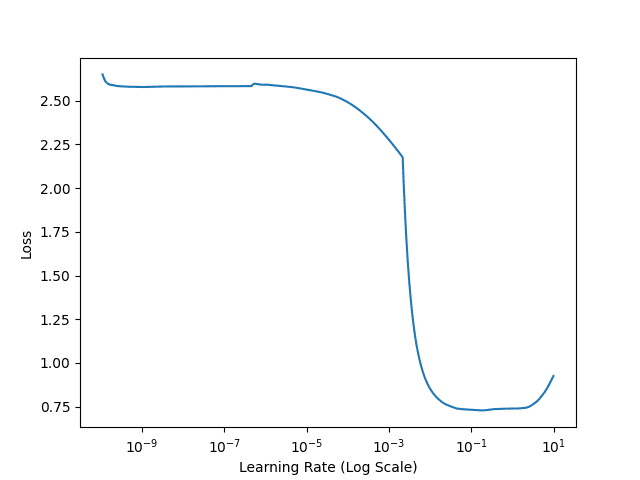Figure 3: Analyzing a deep learning loss vs. learning rate plot to find an optimal learning rate for Keras.

The --lr-find flag instructs our script to utilize the LearningRateFinder class to exponentially increase our learning rate from 1e-10 to 1e+1.

The learning rate is increased after each batch update until our max learning rate is achieved.

Figure 3 visualizes our loss:

• Loss is stagnant and does not decrease from 1e-10 to approximately 1e-6, implying that the learning rate is too small and our network is not learning.
• At approximately 1e-5 our loss starts to decrease, meaning that our learning rate is just large enough that the model can start to learn.
• By 1e-4 and 1e-3 loss is dropping rapidly, indicating that this is a “sweet spot” where the network can learn quickly.
• Just after 1e-2 there is a tiny increase in loss, implying that our learning rate may soon be too large.
• At 1e-1 we see a larger jump, again, indicating that the learning rate is too large.
• And by the time we reach 1e+1, our loss has exploded (the learning rate is far too large).

Based on this plot, we should choose 1e-5 as our base learning rate and 1e-2 as our max learning rate — these values indicate a learning rate just small enough for our network to start to learn, along with a learning rate that this is large enough for our network to rapidly learn, but not so large that our loss explodes.

Be sure to refer back to Figure 2 if you need help analyzing Figure 3.

### Training the entire network architecture

If you haven’t yet, go back to our config.py file and set MIN_LR = 1e-5  and MAX_LR = 1e-2, respectively:

From there, execute the following command: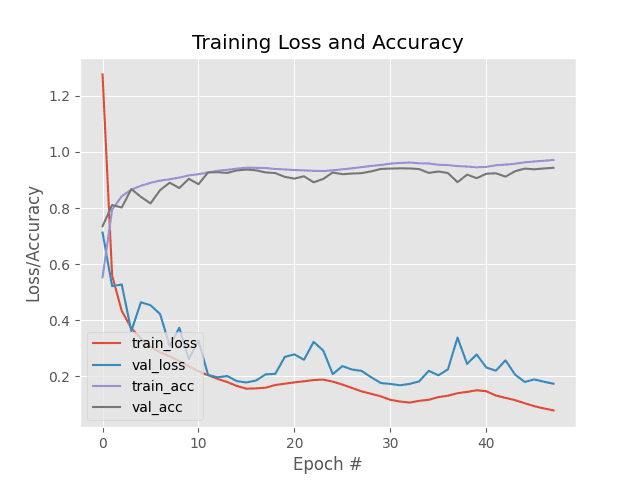Figure 4: Our one and only experiment’s training accuracy/loss curves are plotted while using the min/max learning rate determined via our Keras learning rate finder method.

After training completes we are obtaining 95% accuracy on the Fashion MNIST dataset.

Again, keep in mind that we only ran one experiment to tune the learning rates to our model and we’re over 95% accurate in our predictions!

Our training history is plotted in Figure 4. Notice the characteristic “waves” from a triangular Cyclical Learning Rate policy — our training and validation loss gently rides the waves up and down as our learning rate increases and decreases.

Speaking of the Cyclical Learning Rate policy, let’s look at how the learning rate changes during training: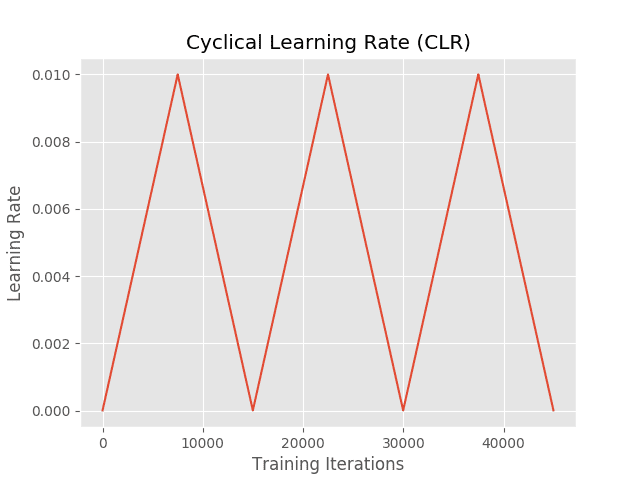Figure 5: A plot of our cyclical learning rate schedule using the “triangular” mode. The min/max learning rates were determined with a Keras learning rate finder.

Here we complete three full cycles of the triangular policy, starting at an initial learning rate of 1e-5, increasing to 1e-2, and then decreasing back to 1e-5 again.

Using a combination of Cyclical Learning Rates along with our automatic learning rate finder implementation, we are able to obtain a highly accurate model in only a single experiment!

You can use this combination of CLRs and automatic learning rate finder in your own projects to help you quickly and effectively tune your learning rates.Figure 6: My deep learning book is the go-to resource for deep learning students, developers, researchers, and hobbyists, alike. Use the book to build your skillset from the bottom up, or read it to gain a deeper understanding. Don’t be left in the dust as the fast paced AI revolution continues to accelerate.

Today’s tutorial introduced you to an automatic method to find learning rate parameters for Cyclical Learning Rates using Keras.

If you’re looking for more of my tips, suggestions, and best practices when training deep neural networks, be sure to refer to my book, Deep Learning for Computer Vision with Python.

Inside the book I cover:

1. Deep learning fundamentals and theory without the unnecessary mathematical fluff. I present the basic equations and back them up with code walkthroughs that you can implement and easily understand.
2. More details on learning rates (and how a solid understanding of the concept impacts your deep learning success).
3. How to spot underfitting and overfitting on-the-fly and how to checkpoint your models with a custom callbacks.
4. My tips/tricks, suggestions, and best practices for training CNNs.

Besides content on learning rates, you’ll also find:

• Super practical walkthroughs that present solutions to actual, real-world image classification, object detection, and instance segmentation problems.
• Hands-on tutorials (with lots of code) that not only show you the algorithms behind deep learning for computer vision but their implementations as well.
• A no-nonsense teaching style that is guaranteed to help you master deep learning for image understanding and visual recognition.

## Summary

In this tutorial you learned how to create an automatic learning rate finder using the Keras deep learning library.

This automatic learning rate finder algorithm follows the suggestions of Dr. Leslie Smith in their 2017 paper, Cyclical Learning Rates for Training Neural Networks (but the method itself wasn’t popularized until Jermey Howard suggested that fast.ai users utilize it).

The primary contribution of this guide is to provide a well-documented Keras learning rate finder, including an example of how to use it.

You can use this learning rate finder implementations when training your own neural networks using Keras, enabling you to:

1. Bypass 10s to 100s of experiments tuning your learning rate.
2. Obtain a high accuracy model with less effort.

Make sure you use this implementation when exploring learning rates with your own datasets and architectures.

I hope you enjoyed my final post in a series of tutorials on finding, tuning, and scheduling learning rates with Keras!If you would like to download the code and images used in this post, please enter your email address in the form below. Not only will you get a .zip of the code, I’ll also send you a FREE 17-page Resource Guide on Computer Vision, OpenCV, and Deep Learning. Inside you'll find my hand-picked tutorials, books, courses, and libraries to help you master CV and DL! Sound good? If so, enter your email address and I’ll send you the code immediately!

### 35 Responses to Keras Learning Rate Finder

1.Tien Su August 5, 2019 at 12:23 pm #

It’s so useful!
Thank you very much!

•Adrian Rosebrock August 6, 2019 at 12:43 pm #

You are welcome, I’m glad you enjoyed it!

2.Umut August 5, 2019 at 12:52 pm #

What about regression problems? Is it applicable for function approximation type regression problems? Can it properly use with LSTM type architectures?
Thank you

•Adrian Rosebrock August 6, 2019 at 12:43 pm #

I haven’t tried it with regression problems but it should work. Give it a try and see!

•Umut August 11, 2019 at 4:53 pm #

Yes, it works but all the regression cases I did not see any improvement and contribution.

3.Rémi C August 6, 2019 at 10:53 am #

Thanks a lot.

•Adrian Rosebrock August 6, 2019 at 12:43 pm #

You are welcome!

4.Nel August 6, 2019 at 11:21 am #

Thanks for this tutorial. Just to make sure, can we find learning rate for other optimizer like Adam in the same way?

•Adrian Rosebrock August 6, 2019 at 12:43 pm #

Exactly. This method can be used for Adam, SGD, RMSprop, etc.

5.Hassan August 7, 2019 at 2:16 am #

Thanks a lot.

•Adrian Rosebrock August 7, 2019 at 12:18 pm #

You are welcome!

6.Rémi C August 7, 2019 at 4:02 am #

I have a question about ImageDataGenerator :
Can we split them (testX testY, trainx trainy) them in any sort ?

•Adrian Rosebrock August 7, 2019 at 12:17 pm #

What do you mean by “in any sort”?

7.tony August 7, 2019 at 8:03 am #

I would like to use your method in my research, Is there any copyrights I should obey?
How can I cite this method?

•Adrian Rosebrock August 7, 2019 at 12:17 pm #

You can use the methods in your research but make sure you cite Leslie Smith’s paper and this tutorial. You can find instructions on how to cite my tutorials here.

8.Niloufar August 8, 2019 at 9:13 am #

Thanks dear Adrian. Just one question: in line 42 and 43 you added number of channel for training purposes, can you explain why we need that? In my model as I have masking+lstm for classification, 4D input is not acceptable as lstm input so how should I solve this problem?

Thanks

•Adrian Rosebrock August 16, 2019 at 6:03 am #

The added dimension is the “batch dimension”. I don’t have any tutorials on LSTMs yet but I’ll likely cover them later this year.

9.Jonathan Mackenzie August 9, 2019 at 5:52 am #

Nice, I did something similar a few months ago where you call the LR finder whenever loss stops decreasing. https://github.com/JonnoFTW/keras_find_lr_on_plateau

Another good tip is to use exponential smoothing on the loss plot.

•Adrian Rosebrock August 16, 2019 at 5:59 am #

Thanks for sharing!

10.JJ August 9, 2019 at 4:00 pm #

I didn’t have time to train for 48 epochs so I cut it down to 6 epochs.

I then tried training it with Adam and no CLR callbacks for 6 epochs as well and ended up with much higher accuracy. In fact, I had already gotten better results with a single epoch of Adam compared to 6 epochs of SGD with CLR using your default settings (except for 6 epochs instead of 48.)

I’ve played around with various CLR implementations in Keras for a while but rarely seem to achieve better accuracy and F1 scores compared to Adam, at least with a small number of epochs.

On the other hand I’m a heavy user of Fastai and they seem to use CLR by default. I haven’t played around with replacing CLR + SGD with Adam in their models though my Keras + Adam often seems to lag behind Fastai + CLR in both F1 and training speed when training on the same dataset.

•Adrian Rosebrock August 16, 2019 at 5:58 am #

In my experience I find that CLR works best with SGD but can absolutely still work well with other optimizers (Adam, RMSprop, etc.). To determine which optimizer and learning rates are best I recommend doing exactly what you have done — run experiments and let the empirical results guide you further.

Secondly, Adam is very much designed to help achieve higher accuracy faster but that doesn’t always mean that it will obtain higher accuracy overall — that is entirely dependent on your dataset and your project (that is why you need to run multiple experiments).

11.Rémi C August 23, 2019 at 6:08 am #

Hello !
I used your code in tensorflow 2, and wanted to know if the LRfidner is usable with tf.records?
I have trouble using it rigth now, with shapes errors.

•Adrian Rosebrock August 23, 2019 at 1:24 pm #

I have not tested it with “tf.records”. My assumption is probably not. You would need to edit the `iterClasses` set to include whatever the name of the “tf.records” data iterator is.

12.Rémi C August 29, 2019 at 7:41 am #

Indeed it worked perfectly ! Im now working on CLR for object detection

•Adrian Rosebrock September 5, 2019 at 10:01 am #

Awesome, I’m glad it worked for you Rémi!

13.Varun Anand October 10, 2019 at 5:43 am #

Hey Adrian! Thank you for this amazing tutorial!

I am training ResNet50 on CIFAR10, and I’m using CLR for the learning rate schedule. However, after about 15 epochs, learning tends to stagnate no matter what I do.
So I tried this:
I serialized the weights after epoch 15 and ran the learning rate finder again initializing the model with these weights. I did this in the hope of finding better values for the base and max lr to continue training from epoch 15. But, the Loss vs LR graph that I get is even more inconclusive. There isn’t a clear dip in the loss. Instead, it just oscillates until a value (1e-3) beyond which, it shoots up.

I have no idea where I’m going wrong…

14.barry October 22, 2019 at 3:51 am #

Hi,which ways of CLR and decay schedules is the best way?How do I choose the ways of CLR and decay schedules

•Adrian Rosebrock October 25, 2019 at 10:10 am #

Hey Barry — this tutorial covers how to use the learning rate finder to find appropriate learning rates. You can then use them with CLR, as we did here, or use the largest reasonable learning rate found via our learning rate finder and use that as the initial learning rate for the decay schedule.

15.Bardia November 5, 2019 at 1:45 pm #

Thank you for your great three-part series on tuning learning rate. They were really helpful to me.

Since you said that normally 3 to 5 epoch is okay for finding the optimal learning rate, is it better to change
“epochs = int(np.ceil(sampleSize / float(stepsPerEpoch)))”
to “epochs = 3” or “epochs = 5”
if “epochs = int(np.ceil(sampleSize / float(stepsPerEpoch)))” is better, what is a good number for “sampleSize” for a different model and dataset?
Also, is “stopFactor = 4” okay for a different problem?

16.Alessandro November 19, 2019 at 12:24 pm #

Hi Adrian, very cool tutorial! I have only one doubt related to the data loading, as you load all the images in a numpy array in advance. What if the data are too much? is there a way to tell the model to load only a batch of data, or to load images one by one, during training?
thanks a lot

•Adrian Rosebrock November 21, 2019 at 8:54 am #

You could use Keras’ ImageDataGenerator class and flow_from_directory function OR you could serialize the dataset in HDF5 format and then build a generator to yield batches from the dataset. I cover how to do that in Deep Learning for Computer Vision with Python.

17.Zubair Ahmed November 24, 2019 at 12:48 pm #

Nice tutorial, does CyclicLR work when you’re loading a model from checkpoint or do you need to set new learning rate using set_value() after loading the checkpoint?

I tried setting the callback CyclicLR after loading from checkpoint but it doesn’t seem to take effect.

Any suggestions, I’m using TF 2.0

•Adrian Rosebrock December 5, 2019 at 10:58 am #

This method will work regardless of whether you’re training from scratch or starting from a checkpoint.

Secondly, keep in mind that this method is testing various learning rates — it doesn’t matter what your previous learning rate is.

18.Ron Cohen December 2, 2019 at 5:14 pm #

Hey Adrian, thanks for posting this (and all of your posts). Your code includes saving weights from an “initialized model” and then restoring them after the learning rate is determined. Does this mean that this learning rate finder technique is intended for models that have already been trained (e.g. up to a learning plateau) or does it also work before any training is done (in which case there is no need to restore prior model weights)?

•Adrian Rosebrock December 5, 2019 at 10:31 am #

You can use this learning rate finder for models that are freshly initialized or have already been trained. We simply keep a copy of the model weights to reset them, that way they are “as they were” before you ran the learning rate finder.